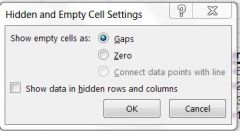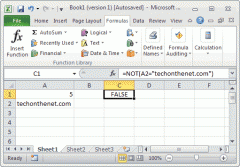• Shuffle
Toggle On
Toggle Off
• Alphabetize
Toggle On
Toggle Off
• Front First
Toggle On
Toggle Off
• Both Sides
Toggle On
Toggle Off
Toggle On
Toggle Off
Front

### How to study your flashcards.

Right/Left arrow keys: Navigate between flashcards.right arrow keyleft arrow key

Up/Down arrow keys: Flip the card between the front and back.down keyup key

H key: Show hint (3rd side).h key

A key: Read text to speech.a keyPlay buttonPlay buttonProgress

1/47

Click to flip

### 47 Cards in this Set

• Front
• Back
 Total from multiple sheets =sum(then click in cells to add uphit EnterIf many worksheets type =sum( then click first cell in 1st worksheet to add to formula then SHIFT and click last worksheet tab then Enter Select entire worksheet CTRL + A + A if active cell is w/in rangeCTRL + A if active cell is not in a range Select range CTRL + A when active cell is w/in range syntax for workbook name when working with multiple workbooks [example] syntax for named worksheet if includes spaces single quotes `example' syntax for cell reference working with multiple worksheets ! exclamation pointexample: =sum(Sheet3!a3,Sheet5!g7) Quick Analysis Tool options FormattingChartsTotals (sum = grand total)TablesSparklines Create sparkline Select cellInsertChoose type (Line, Column, Win/Loss)Select data rangeEnter Sparkline Tool Design tab options Edit data (change how hidden/empty cells are treated)Change type (Line, Column, Win/Loss)Show - high point, low point, Negative points, First point, last pointChange style Change sparkline or marker colors Change axis, group/ungroup, clear Win/Loss When have negative and positive numbersTo add contrast Change result of formula into a value Click in cell with formulaF2 then F9 Edit sparkline data optionsshow empty cells as gaps, zeros, connect data points with linesShow data in hidden rows/columns Data consolidate - definition when worksheets have different column/row headers (e.g. people, products, etc.). Make sure names spelled same across all sheets or they'll be treated as different data elementscan do:totalscountaveragemaxmincount #sstandard deviationetc. Data consolidation - how Data tab - Consolidate buttonSelect range with mouseClick AddRepeat as necessary with other data rangesSelect labels - top and leftSelect "create links to source data"Enter Data consolidation - hierarchy outline 1 = highest (collapsed) level2 = expands data3 = view entire worksheetclick + to expand and - to collapse Keyboard shortcut to reveal all formulas CTRL ` Subtotals Click inside data rangeData tab -> SubtotalSelect which labeled columns to add subtotals to (eg. at end of every year)Functions available: Sum, Count, Ave, Max, Min, Product, Count numbers, StdDev, StdDevp, Var, Varpalso creates a grand total Group data Select data rangeData tab -> ounline group -> group -> choose rows or columnsadd level to outline/hierarchy that can be hidden (collapsed with -) or show (expand with +) copy data from subtotaled range Select rangeGo To (CTRL + G) -> Special -> by criterion -> Visible cells onlyCTRL + C to copySelect location CTRL + V to paste VLOOKUP Doesn't calculate - just geographic identity v for Vertical - Hlookup for horizontalRANGE lookup = default (#1) - only 3 arguments = ascending (A-Z) order sortExact Match = #2 special form - 4 arguments - no sorting necessary=VLOOKUP(lookup value, table array, column index # of data to be returned as answer to query,range_lookup)example: =VLOOKUP(K2,F2:H10,3) Range lookup optional - default is TRUE - TRUE assumes the first column in the table is sorted either numerically or alphabetically, and will then search for the closest value. FALSE searches for the exact value in the first column. VLOOKUP Type 2 exact match - 4 arguments=VLOOKUP(lookup_value, table_name or data range, column_index_number,FALSE)FALSE because override default "approximate" lookup (TRUE) Nested Formulas Up to 64 order = deeply nested 1st then work out to leftorder indicated by color (1st is black)pairs indicated by paranthesesABSFINDLEFTROWCOLUMN ABS formula =ABS(cell) - removes sign from number ex: \$,-,%, etc.example: =abs(sum(data range)) Entermake sure to include correct number of closing parentheses If get error- pop up window shows suggested formula format Autosum speed key / shortcut ALT = Display formula Function key F2 when in cell with formula =FIND =FIND(useful in nested formulasexample: =FIND("-",b4) Displays character position of character included in formula within cell reference =LEFT Displays/extracts all characters to left of column index # included in formula=LEFT(E2,3) = fixed positionNESTED example for non-fixed position: =LEFT(E2,FIND("-",E2)) - extracts all characters to left of dash -aaa-aa-a- =ROW Tells what row cell is in example: =ROW(G4) = row #4 =COLUMN Provides column index # for cellexample: =COLUMN(E3) = column #5 R1C1 cell reference style uses row index # and column index # to reference cellsexample: A10 becomes R10C1 (row 10, column 1)can be useful when using more than 26 columns - such as cell AX1 = column AX, row 1 = in RICI format it's R1C50To change to RICI format, FILE tab -> Options -> Formulas -> RICI Conditional Logic =IF=AND=OR=NOT=IFERROR=SUMIF=COUNTIF=AVERAGEIF=SUMIFS=COUNTIFS=AVERAGEIFS =IF means "if true, then X, otherwise Y"=IF(A1=-100,"HURRAY!","NO GOOD")so, if statement true- display second argument, if not true display third argument=IF(A2<>-200,0,"") so if data in cell A2 is not equal to -200, display a zero, otherwise display a blank cellCan display text string, blank cell, math operation, or formula=IF(A2<>-200,0,ABS(SUM(H2:H5)))so if data in A2 is not equal to -200, display 0, otherwise display Absolute Value of sum total of range H2:H5Often nested with VLOOKUP formulas - test X and if true look up Y or if false look up Z Nested IF test 2 statements=IF(A3<>-300,"xxx",if(A3=-300,"A true answer",0))so if A3=-300 display "A true answer", or if not equal to -300 display xxx. Compatibility Mode older files before Office 2007 - some stuff won't displaylook in file title to see if opened in compatibility modeSome Excel 2013 features won't work- features will be greyed out, e.g. Flash FillConvert = FILE tab -> Info -> Convert -> rename or replace Can't widen columns Turn wrap text off Formula wizard CTRL + A once formula begunOR use FX button in formula bar to select from list of recently used or via categories =AND =AND(A2>20000,A2>30000)BOTH logical tests MUST BE TRUE to display TRUE - otherwise FALSE =OR =OR(A2>20000,A2<30000) Only one logical test has to be TRUE to result in TRUE - so only FALSE is BOTH FALSE =NOTEXCLUDESDisplays OPPOSITE of logical test result=NOT(A2>100000) =IFERROR Tells Excel what to do if errors=5/0 if lots of errors- allows to display something other than #DIV! etc=IFERROR(A1/B2, 0) = so divide A1 by B2 but if produces error then display alternative value =SUMIF =SUMIF(range, criteria) So sum all data selected that meet certain criteriaexample: =SUMIF(A2:A12,"<20") means sum data in range A2 thru A12 that is less than 20 =SUMIFS Sum all data selected that meet both criteria=SUMIFS(B2:E2,B4:E4,">=75",B3:E3,"<40%") for the four days, add the total hours of sunshine (range B2:E2) WHEN the average temperature (range B4:E4) was at least 75 degrees Fahrenheit and the average humidity (range B3:E3) was less than 40%.use CTRL + A to get formula wizard =COUNTIF count selected cells that meet criteria =COUNTIFS count selected cells that meet both criteria=COUNTIFS(B4:E4,">=75",B3:E3,"<40%")For the four days count only those days when the average temperature (B4:E4) was at least 75 degrees Fahrenheit and the average humidity (B3:E3) was less than 40%. =AVERAGEIF Average selected cells that meet criteria =AVERAGEIFS Average selected cells that meet both critera=AVERAGEIFS(B2:E2,B4:E4,">=75",B3:E3,"<40%")For the four days calculate the average hours of sunshine (B2:E2) when the average temperature (B4:E4) was at least 75 degrees Fahrenheit and the average humidity (B3:E3) was less than 40%. Wildcard symbol * - replace characters to right of text string